# Python 基础知识入门（三）

• 2023-02-24
北京
• 本文字数：1654 字

阅读完需：约 5 分钟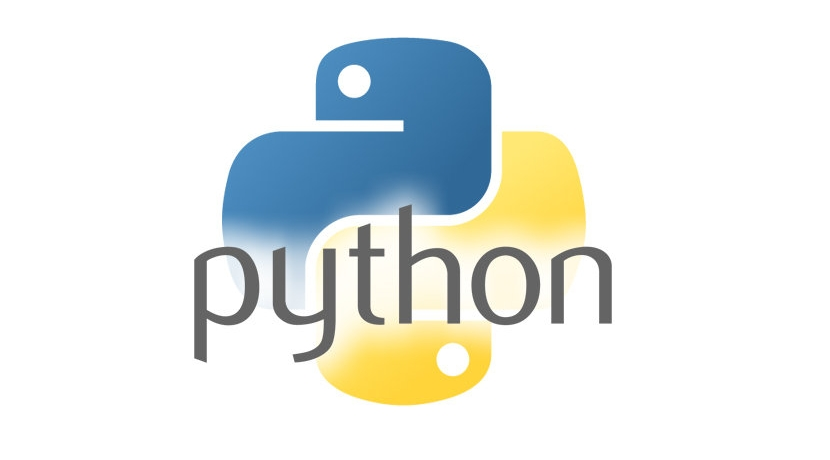### 一、元组类型

#### 1.元组函数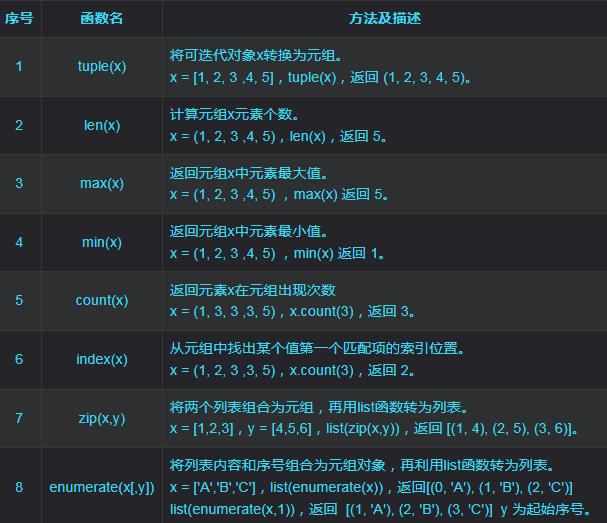#### 2.元组切片

x = (1, 2, 3, 4, 5, 6)print(x)             # 3                     查找元组下标为2的元素print(x[-2])            # 5                     查找元组倒数第2个元素print(x[:])             # (1, 2, 3, 4, 5, 6)    查找元组下标为0到末尾的元素print(x[::2])           # (1, 3, 5)             查找元组下标0到末尾间隔为2的元素print(x[:5])            # (1, 2, 3, 4, 5)       查找元组下标为0到5的元素print(x[1:])            # (2, 3, 4, 5, 6)       查找元组下标为1到末尾的元素print(x[1:-2])          # (2, 3, 4)             查找元组下标1的到倒数第2个间的元素print(x[-5:-3])         # (2, 3)                查找元组倒数第5到倒数第3间的元素

#### 3.元组运算

x = (1, 2, 3)y = (4, 5, 6) print(len(x))       # 3                     计算元组元素个数print(x + y)        # (1, 2, 3, 4, 5, 6)    元组 x 和元组 y 拼接print(x*2)          # (1, 2, 3, 1, 2, 3)    复制指定系数元组 xprint(5 in y)       # True                  判断 5 是否在元组 x 内print(5 not in y )  # False                 判断 5 是否不在元组 x 内print(x!=y)         # True                  判断元组 x 和 y 是否相等

### 二、字典类型

字典是由大括号 {} 包裹所有元素。字典的每个元素是键值对，中间用英文冒号连接（:）如 {'name': '漫步桔田'}，其中我们把 'name' 叫 键（key），' 漫步桔田' 叫 值（value）。

#### 1.字典函数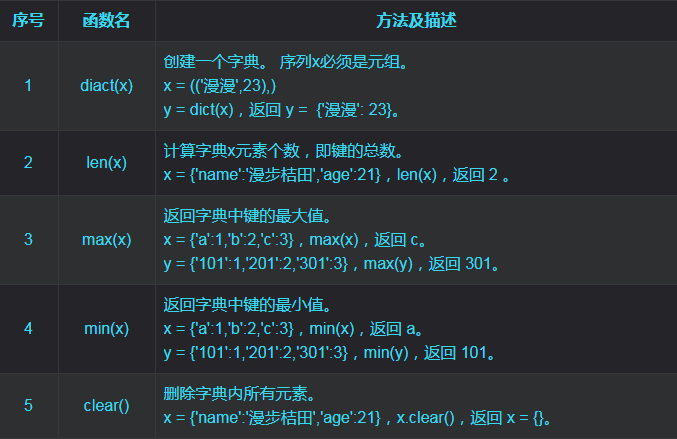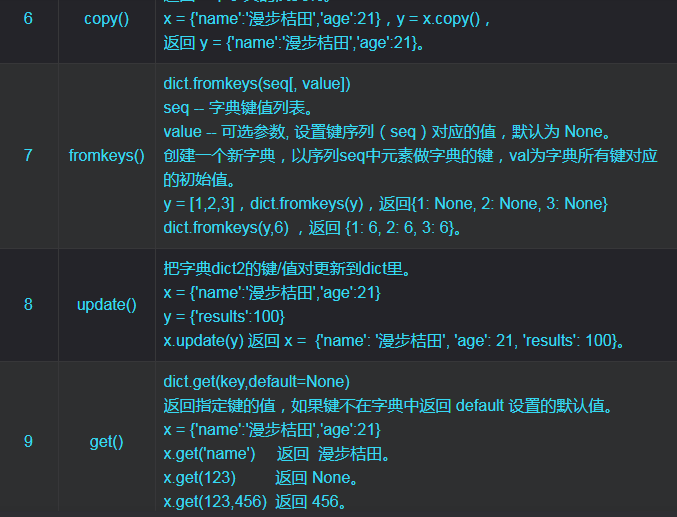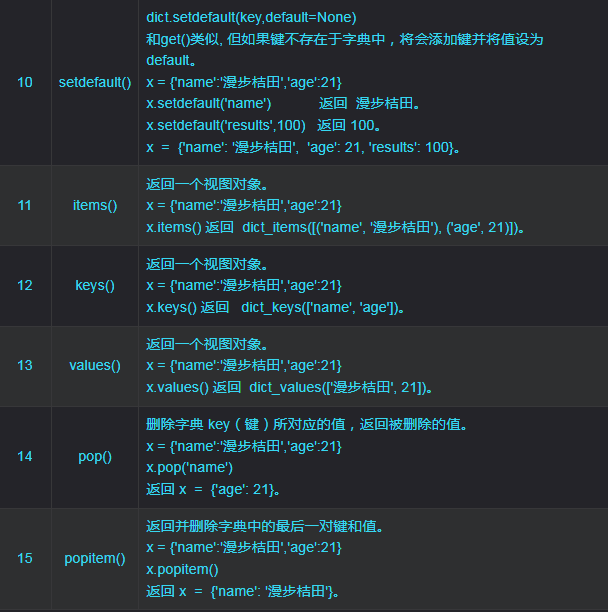#### 2.字典操作

# 字典创建x = dict(name ='漫步桔田',age = 21)print(x)               # {'name': '漫步桔田', 'age': 21}  name = ['漫漫','桔桔']age = [23,21]x = dict(zip(name,age))print(x)               # {'漫漫': 23, '桔桔': 21} # 字典增加x = {'name':'漫步桔田','age':21}x['scores'] = 100print(x)               # {'name': '漫步桔田', 'age': 21, 'scores': 100}  # 字典删除x = {'name':'漫步桔田','age':21}del x['age']print(x)               # {'name': '漫步桔田'}  # 字典修改x = {'name':'漫步桔田','age':21}x['age'] = 25print(x)               # {'name': '漫步桔田', 'age': 25}  # 字典查询x = {'name':'漫步桔田','age':21}print(x['age'])        # 21  # 字典嵌套x = {}x['name'] = [1,2,3]   print(x)              # {'name': [1, 2, 3]}print(x['name'])   # 2 嵌套取值   # 字典判断x = {'漫漫': 23, '桔桔': 21}  print('漫漫' in x)     # True  判断字典键中是否包含'漫漫'print('小漫' in x)     # False 判断字典键中是否包含'小漫'

### 三、集合类型

#### 1.集合函数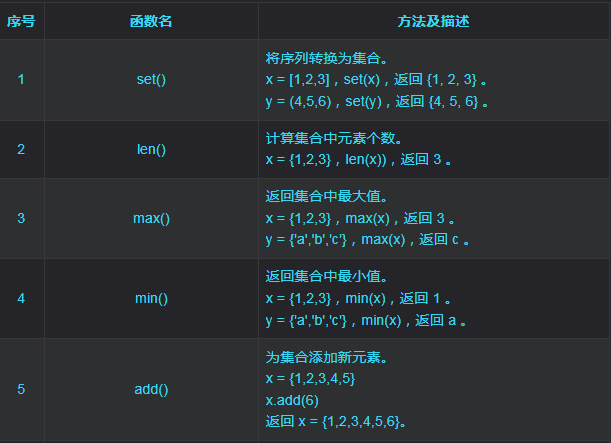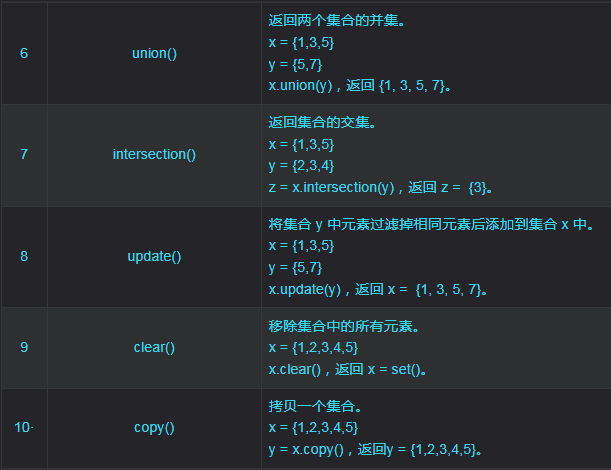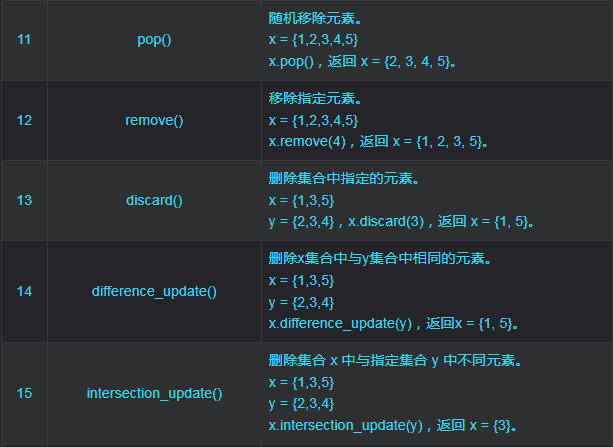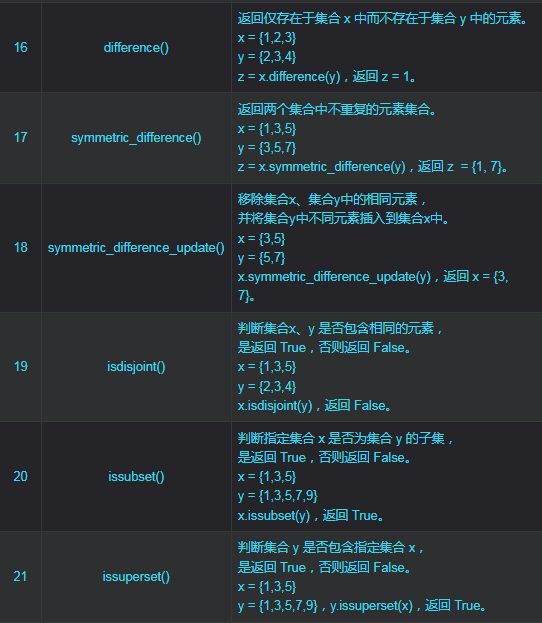#### 2.集合操作

x = {1,2,3}print(2 in x)         # True 判断元素 2 是否在集合 x 中 x = [1,1,2,3,5,5]print(list(set(x)))   # [1, 2, 3, 5] 列表、元组去重 x = {1,3,5}y = {2,3,4} print(x-y)            # {1, 5}          #差集：返回一个新集合，包括集合 x 中与集合y不同元素。 print(x|y)            # {1, 2, 3, 4, 5} #并集：返回一个新集合，包括集合 x 和集合 y 中所有元素。 print(x&y)            # {3}             #交集：返回一个新集合，仅包括集合 x 和 y 中相同的元素。 print(x^y)            # {1, 2, 4, 5}    #补集：返回一个新集合，仅包括集合 x 和 y 中的不同元素。

CSDN 博主「漫步桔田」原文链接：https://blog.csdn.net/u013157570/article/details/127784795## 评论